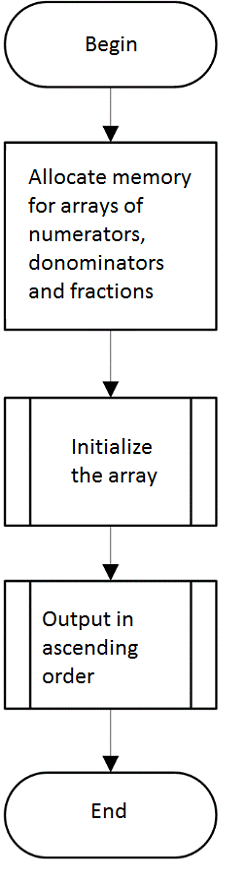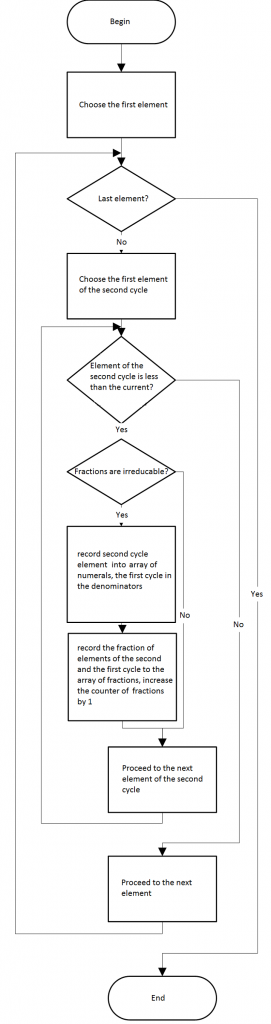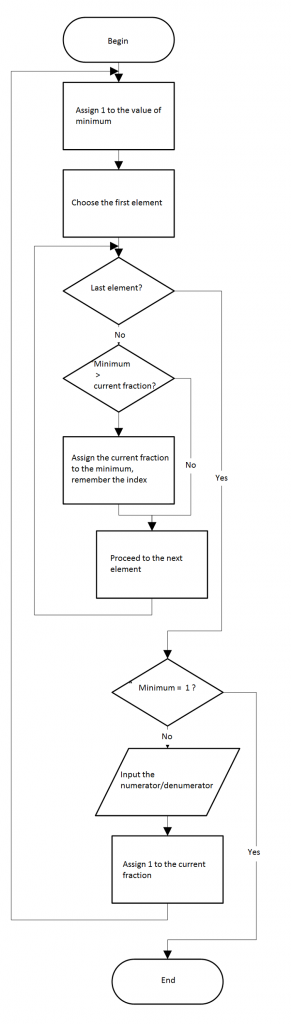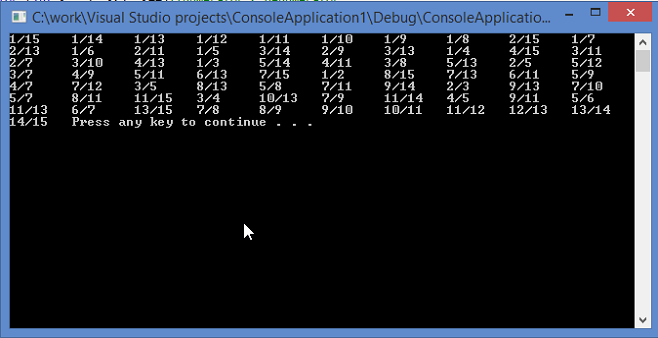# Assignment Samples: The One About Pointers

Output in ascending order all regular irreducible fractions ranging from 0 to 1, denominators of which do not exceed 15. The numerator and denominator should be represented as pointers to type int.

Block-schemes of the solution:C++ implementation:

```#include<iostream>

using namespace std;

int n;//amount of fractions
int lcd(int a, int b)//find NSD of two numbers; Evclid algorithm
{
int t;
while (b != 0)
{
t = b;
b = a%b;
a = t;
}
return a;
}

void init(int*up, int*down, float*fraction, int num)//initialize the array
{
for (int i = 1; i <= num; i++)//cycle for fractions
for (int k = 1; k<i; k++)//numerator < denumerator
if (lcd(i, k) == 1)//irreducable
{
up[n] = k;//denumerator
down[n] = i;//numerator
fraction[n] = (float)up[n] / (float)down[n];//calculate value of fraction
n++;//number of fractions
}
}
void output(int*up, int*down, float*fraction, int num)//output in ascending order
{
for (;;)//find min and output
{
float min = 1;//due to the condition that all fractions are less than 1
int number;//number of element of array
for (int i = 0; i<n; i++)//cycle for fractions { if (min>fraction[i])
{
min = fraction[i];//find min
number = i;//remember number of min
}
}
if (min == 1) break;//fractions are over
cout << up[number] << '/' << down[number] << '\t';//output min
fraction[number] = 1;//avoid repetition
}
}

void main()
{
int num = 15;//max denumerator
int *up = new int[num*num];//dynamic array of numerators
int *down = new int[num*num];//dynamic array of denumerators
float *fraction = new float[num*num];//dynamic array of fraction values
init(up, down, fraction, num);
output(up, down, fraction, num);
system("pause");
}
```

ScreenshotsAnalysis of the reliability of the results:

1/15=0.0666667
1/14=0.0714286
1/13=0.0769231
1/12=0.0833333
1/11=0.0909091
1/10=0.1
1/9=0.111111
1/8=0.125
2/15=0.133333
1/7=0.142857
2/13=0.153846
1/6=0.166667
2/11=0.181818
1/5=0.2
3/14=0.214286
2/9=0.222222
3/13=0.230769
1/4=0.25
4/15=0.266667
3/11=0.272727
2/7=0.285714
3/10=0.3
4/13=0.307692
1/3=0.333333
5/14=0.357143
4/11=0.363636
3/8=0.375
5/13=0.384615
2/5=0.4
5/12=0.416667
3/7=0.428571
4/9=0.444444
5/11=0.454545
6/13=0.461538
7/15=0.466667
1/2=0.5
8/15=0.533333
7/13=0.538462
6/11=0.545455
5/9=0.555556
4/7=0.571429
7/12=0.583333
3/5=0.6
8/13=0.615385
5/8=0.625
7/11=0.636364
9/14=0.642857
2/3=0.666667
9/13=0.692308
7/10=0.7
5/7=0.714286
8/11=0.727273
11/15=0.733333
3/4=0.75
10/13=0.769231
7/9=0.777778
11/14=0.785714
4/5=0.8
9/11=0.818182
5/6=0.833333
11/13=0.846154
6/7=0.857143
13/15=0.866667
7/8=0.875
8/9=0.888889
9/10=0.9
10/11=0.909091
11/12=0.916667
12/13=0.923077
13/14=0.928571
14/15=0.933333

All of our assignment samples were completed by our experts including this one about pointers. If you are assigned with a similar task and you are having difficulties with it apply to AssignmentShark for help. We hire well-qualified experts with vast experience. Therefore, you are able to get help even with the most complicated assignments. Whenever you need help contact us. We’ll do our best to provide you with qualified help. Our service is available 24/7. Also, you should know that we keep 100% confidentiality. Look through other assignment samples (the one about Vertex Shader example within this category, for example) and make your final decision right now!